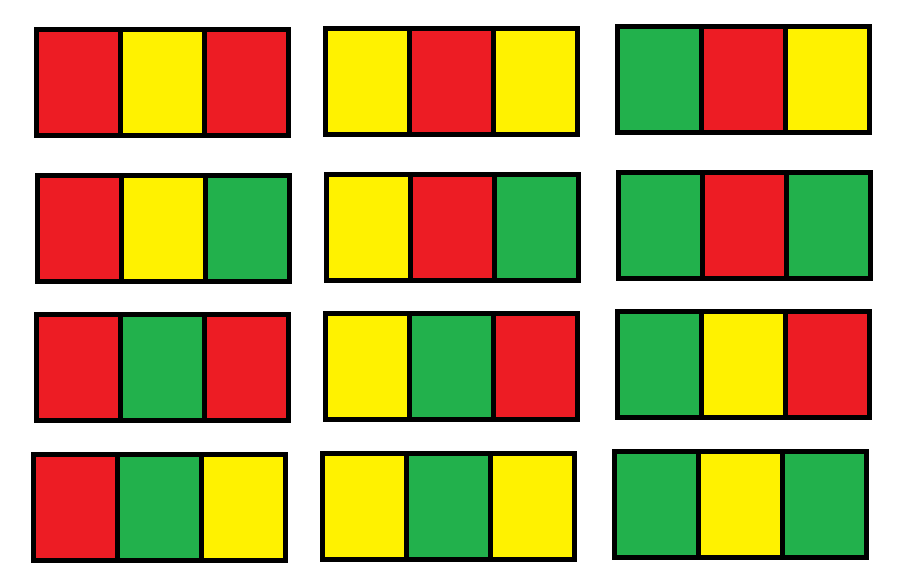# GeetCode Hub

You have a `grid` of size `n x 3` and you want to paint each cell of the grid with exactly one of the three colors: Red, Yellow, or Green while making sure that no two adjacent cells have the same color (i.e., no two cells that share vertical or horizontal sides have the same color).

Given `n` the number of rows of the grid, return the number of ways you can paint this `grid`. As the answer may grow large, the answer must be computed modulo `109 + 7`.

Example 1:```Input: n = 1
Output: 12
Explanation: There are 12 possible way to paint the grid as shown.
```

Example 2:

```Input: n = 2
Output: 54
```

Example 3:

```Input: n = 3
Output: 246
```

Example 4:

```Input: n = 7
Output: 106494
```

Example 5:

```Input: n = 5000
Output: 30228214
```

Constraints:

• `n == grid.length`
• `grid[i].length == 3`
• `1 <= n <= 5000`

class Solution { public int numOfWays(int n) { } }